International
Tables for
Crystallography
Volume F
Crystallography of biological macromolecules
Edited by M. G. Rossmann and E. Arnold

International Tables for Crystallography (2006). Vol. F, ch. 18.5, pp. 412-414   | 1 | 2 |

## Section 18.5.8. Luzzati plots

D. W. J. Cruickshanka*

aChemistry Department, UMIST, Manchester M60 1QD, England
Correspondence e-mail: dwj_cruickshank@email.msn.com

### 18.5.8. Luzzati plots

| top | pdf |

#### 18.5.8.1. Luzzati's theory

| top | pdf |

Luzzati (1952)provided a theory for estimating, at any stage of a refinement, the average positional shifts which would be needed in an idealized refinement to reach. He did not provide a theory for estimating positional errors at the end of a normal refinement.

 (1) His theory assumed that thehad no errors, and that themodel (scattering factors, thermal parameters etc.) was perfect, apart from coordinate errors. (2) The Gaussian probability distribution for these coordinate errors was assumed to be the same for all atoms, independent of Z or B. (3) The atoms were not required to be identical, and the position errors were not required to be small.

Luzzati gave families of curves for R versusfor varying average positional errors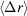for both centrosymmetric and noncentrosymmetric structures. The curves do not depend on the number N of atoms in the cell. They all rise fromat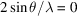to the Wilson (1950)values 0.828 and 0.586 for random structures at high. Table 18.5.8.1gives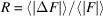as a function of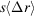for three-dimensional noncentrosymmetric structures.

 Table 18.5.8.1| top | pdf |as a function ofin the Luzzati model for three-dimensional noncentrosymmetric structures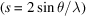RR
0.00 0.000 0.10 0.237
0.01 0.025 0.12 0.281
0.02 0.050 0.14 0.319
0.03 0.074 0.16 0.353
0.04 0.098 0.18 0.385
0.05 0.122 0.20 0.414
0.06 0.145 0.25 0.474
0.07 0.168 0.30 0.518
0.08 0.191 0.35 0.548
0.09 0.214 0.586

In a footnote (p. 807), Luzzati suggested that at the end of a normal refinement (with R nonzero due to experimental and model errors, etc.), the curves would indicate an upper limit for. He noted that typical small-molecule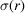's of 0.01–0.02 Å, if used asin the plots, would give much smaller R's than are found at the end of a refinement.

As examples, the Luzzati plots for the two structures of TGF-β2 are shown in Fig. 18.5.8.1. Daopin et al. (1994)inferred average's around 0.21 Å for 1TGI and 0.23 Å for 1TGF.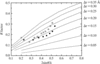Figure 18.5.8.1 | top | pdf |Luzzati plots showing the refined R factor as a function of resolution for 1TGI (solid squares) and 1TGF (open squares) (Daopin et al., 1994).

Of the three Luzzati assumptions summarized above, the most attractive is the third, which does not require the atoms to be identical nor the position errors to be small. For proteins, there are very obvious difficulties with assumption (2). Errors do depend very strongly on Z and B. In the high-angle data shells, atoms with large B's contribute neither tonor to, and so have no effect on R in these shells. In their important paper on protein accuracy, Chambers & Stroud (1979)said `the [Luzzati] estimate derived from reflections in this range applies mainly to [the] best determined atoms.'

Thus a Luzzati plot seems to allow a cautious upper-limit statement about the precision of the best parts of a structure, but it gives little indication for the poor parts.

One reason for the past popularity of Luzzati plots has been that the R values for the middle and outer shells of a structure often roughly follow a Luzzati curve. Evidently, the effective averagefor the structure must be decreasing asincreases, since atoms of high B are ceasing to contribute, whereas the proportionate experimental errors must be increasing. This also suggests that the upper limit forfor the low-B atoms could be estimated from the lowest Luzzati theoretical curve touched by the experimental R plot. Thus in Fig. 18.5.8.1the upper limits for the low-B atoms could be taken as 0.18 and 0.21 Å, rather than the 0.21 and 0.23 Å chosen by Daopin et al.

From the introduction ofby Brünger (1992)and the discussion ofby Tickle et al. (1998b), it can be seen that Luzzati plots should be based on a residual more akin tothan R in order to avoid bias from the fitting of data.

The mean positional errorof atoms can also be estimated from theplots of Read (1986, 1990). This method arose from Read's analysis of improved Fourier coefficients for maps using phases from partial structures with errors. It is preferable in several respects to the Luzzati method, but like the Luzzati method it assumes that the coordinate distribution is the same for all atoms. Luzzati and/or Read estimates ofare available for some of the structures in Tables 18.5.7.2and 18.5.7.3. Often, the two estimates are not greatly different.

#### 18.5.8.2. Statistical reinterpretation of Luzzati plots

| top | pdf |

Luzzati plots are fundamentally different from other statistical estimates of error. The Luzzati theory applies to an idealized incomplete refinement and estimates the average shifts needed to reach. In the least-squares method, the equations for shifts are quite different from the equations for estimating variances in a converged refinement. However, Luzzati-style plots of R versuscan be reinterpreted to give statistically based estimates of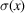.

During Cruickshank's (1960)derivation of the approximate equation (18.5.6.2)forin diagonal least squares, he reached an intermediate equation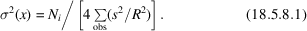He then assumed R to be independent of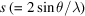and took R outside the summation to reach (18.5.6.2)above.

Luzzati (1952)calculated the acentric residual R as a function of, the average radial error of the atomic positions. His analysis shows that R is a linear function of s andfor a substantial range of, with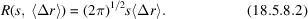The theoretical Luzzati plots of R are nearly linear for small-to-medium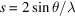(see Fig. 18.5.8.1). If we substitute this R in the least-squares estimate (18.5.8.1)and use the three-dimensional-Gaussian relation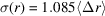, some manipulation (Cruickshank, 1999) along the lines of Section 18.5.6eventually yields a statistically based formula,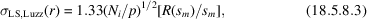where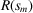is the value of R at some value of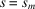on the selected Luzzati curve. Equation (18.5.8.3)provides a means of making a very rough statistical estimate of error for an atom with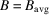(the average B for fully occupied sites) from a plot of R versus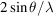.

The corresponding equation involvingis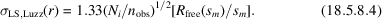#### 18.5.8.3. Comments on Luzzati plots

| top | pdf |

Protein structures always show a great range of B values. The Luzzati theory effectively assumes that all atoms have the same B. Nonetheless, the Luzzati method applied to high-angle data shells does provide an upper limit forfor the atoms with low B. It is an upper limit since experimental errors and model imperfections are not allowed for in the theory.

Low-resolution structures can be determined validly by using restraints, even though the number of diffraction observations is less than the number of atomic coordinates. The Luzzati method, based preferably on, can be applied to the atoms of low B in such structures. As the number of observations increases, and the resolution improves, the Luzzatiincreasingly overestimates the trueof the low-B atoms.

In the use of Luzzati plots, the method of refinement, and its degree of convergence, is irrelevant. A Luzzati plot is a statement for the low-B atoms about the maximum errors associated with a given structure, whether converged or not.

### References

Brünger, A. T. (1992). Free R-value: a novel statistical quantity for assessing the accuracy of crystal structures. Nature (London), 355, 472–475.Google Scholar
Chambers, J. L. & Stroud, R. M. (1979). The accuracy of refined protein structures: comparison of two independently refined models of bovine trypsin. Acta Cryst. B35, 1861–1874.Google Scholar
Cohen, G. H., Sheriff, S. & Davies, D. R. (1996). Refined structure of the monoclonal antibody HyHEL-5 with its antigen hen egg-white lysozyme. Acta Cryst. D52, 315–326.Google Scholar
Cruickshank, D. W. J. (1960). The required precision of intensity measurements for single-crystal analysis. Acta Cryst. 13, 774–777.Google Scholar
Cruickshank, D. W. J. (1999). Remarks about protein structure precision. Acta Cryst. D55, 583–601.Google Scholar
Daopin, S., Davies, D. R., Schlunegger, M. P. & Grütter, M. G. (1994). Comparison of two crystal structures of TGF-β2: the accuracy of refined protein structures. Acta Cryst. D50, 85–92.Google Scholar
Deacon, A., Gleichmann, T., Kalb (Gilboa), A. J., Price, H., Raftery, J., Bradbrook, G., Yariv, J. & Helliwell, J. R. (1997). The structure of concanavalin A and its bound solvent determined with small-molecule accuracy at 0.94 Å resolution. J. Chem. Soc. Faraday Trans. 93, 4305–4312.Google Scholar
Haridas, M., Anderson, B. F. & Baker, E. N. (1995). Structure of human diferric lactoferrin refined at 2.2 Å resolution. Acta Cryst. D51, 629–646.Google Scholar
Ko, T.-P., Day, J., Greenwood, A. & McPherson, A. (1994). Structures of three crystal forms of the sweet protein thaumatin. Acta Cryst. D50, 813–825.Google Scholar
Kobe, B. & Deisenhofer, J. (1995). A structural basis of the interactions between leucine-rich repeats and protein ligands. Nature (London), 374, 183–186.Google Scholar
Luzzati, V. (1952). Traitement statistique des erreurs dans la determination des structures cristallines. Acta Cryst. 5, 802–810.Google Scholar
Read, R. J. (1986). Improved Fourier coefficients for maps using phases from partial structures with errors. Acta Cryst. A42, 140–149.Google Scholar
Read, R. J. (1990). Structure-factor probabilities for related structures. Acta Cryst. A46, 900–912.Google Scholar
Sevcik, J., Dauter, Z., Lamzin, V. S. & Wilson, K. S. (1996). Ribonuclease from Streptomyces aureofaciens at atomic resolution. Acta Cryst. D52, 327–344.Google Scholar
Stec, B., Zhou, R. & Teeter, M. M. (1995). Full-matrix refinement of the protein crambin at 0.83 Å and 130 K. Acta Cryst. D51, 663–681.Google Scholar
Tickle, I. J., Laskowski, R. A. & Moss, D. S. (1998a). Error estimates of protein structure coordinates and deviations from standard geometry by full-matrix refinement of γB- and βB2-crystallin. Acta Cryst. D54, 243–252.Google Scholar
Tickle, I. J., Laskowski, R. A. & Moss, D. S. (1998b). Rfree and the Rfree ratio. I. Derivation of expected values of cross-validation residuals used in macromolecular least-squares refinement. Acta Cryst. D54, 547–557.Google Scholar
Usón, I., Pohl, E., Schneider, T. R., Dauter, Z., Schmidt, A., Fritz, H.-J. & Sheldrick, G. M. (1999). 1.7 Å structure of the stabilized REIV mutant T39K. Application of local NCS restraints. Acta Cryst. D55, 1158–1167.Google Scholar
Wilson, A. J. C. (1950). Largest likely values for the reliability index. Acta Cryst. 3, 397–398.Google Scholar